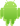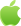# python中pyg2plot如何使用？

## 最佳答案：

PyG2Plot是一款基于Python的数据可视化库，它提供了一系列强大的图表类型和工具，可以帮助用户快速创建美观、交互性强的数据可视化图表。本文将从多个角度分析PyG2Plot的使用，包括安装、基本使用、图表类型、数据格式、主题等方面，帮助读者更好地了解和使用PyG2Plot。

```python

!pip install pyg2plot

```

```python

import pyg2plot

print(pyg2plot.__version__)

```

PyG2Plot的使用非常简单，只需要引入相应的模块，创建图表对象，然后传入相应的数据和配置即可。以下是一个简单的例子，展示如何使用PyG2Plot创建一个柱状图：

```python

from pyg2plot import Plot

data = [

{"x": "A", "y": 10},

{"x": "B", "y": 20},

{"x": "C", "y": 30},

{"x": "D", "y": 40},

{"x": "E", "y": 50},

]

config = {

"xField": "x",

"yField": "y",

"columnStyle": {"fill": "#1890ff"},

"label": {"position": "middle", "style": {"fill": "#fff"}},

}

plot = Plot("Column")

plot.set_data(data)

plot.set_config(config)

plot.render_notebook()

```

PyG2Plot支持多种图表类型，包括柱状图、折线图、饼图、散点图、雷达图等。以下是一些常用的图表类型及其创建方式：

1.柱状图

```python

plot = Plot("Column")

plot.set_data(data)

plot.set_config(config)

plot.render_notebook()

```

2.折线图

```python

plot = Plot("Line")

plot.set_data(data)

plot.set_config(config)

plot.render_notebook()

```

3.饼图

```python

plot = Plot("Pie")

plot.set_data(data)

plot.set_config(config)

plot.render_notebook()

```

4.散点图

```python

plot = Plot("Scatter")

plot.set_data(data)

plot.set_config(config)

plot.render_notebook()

```

5.雷达图

```python

plot.set_data(data)

plot.set_config(config)

plot.render_notebook()

```

PyG2Plot支持多种数据格式，包括列表、字典、pandas数据框等。以下是一些常用的数据格式及其创建方式：

1.列表

```python

data = [

{"x": "A", "y": 10},

{"x": "B", "y": 20},

{"x": "C", "y": 30},

{"x": "D", "y": 40},

{"x": "E", "y": 50},

]

```

2.字典

```python

data = {"x": ["A", "B", "C", "D", "E"], "y": [10, 20, 30, 40, 50]}

```

3.pandas数据框

```python

import pandas as pd

df = pd.DataFrame({"x": ["A", "B", "C", "D", "E"], "y": [10, 20, 30, 40, 50]})

data = df.to_dict("records")

```

PyG2Plot支持多种主题，可以根据需求选择不同的主题来美化图表。以下是一些常用的主题及其使用方式：

1.默认主题

```python

from pyg2plot import set_theme

set_theme("default")

```

2.暗黑主题

```python

from pyg2plot import set_theme

set_theme("dark")

```

3.粉色主题

```python

from pyg2plot import set_theme

set_theme("pink")

```2022-03-29 更新iPhone下载Android下载